# Cauchy operator

(diff) ← Older revision | Latest revision (diff) | Newer revision → (diff)

of a system of ordinary differential equations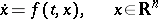The operator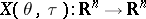, depending on two parametersand, which, given the value of any solution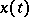of the system

at the point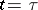, gives the value of that solution at the point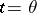: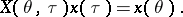If

is a linear system, i.e.(2)

where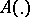is a mapping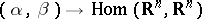(or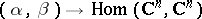), summable over every interval, then for any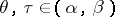the Cauchy operator is a non-singular linear mapping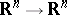(or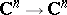), and for any, it satisfies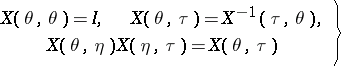(3)

and the inequality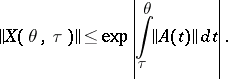(The equations (3) are also valid for a non-linear system

satisfying the conditions of the existence and uniqueness theorem for solutions of the Cauchy problem, with the necessary stipulations concerning the domains of definition of the operators figuring therein.) The general solution of the systemwhere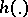is a mappingsummable on every interval, is described in terms of the Cauchy operator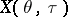of the system (2) by the formula of variation of constants: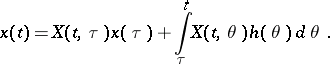The Cauchy operator of the system (2) satisfies the Liouville–Ostrogradski formula: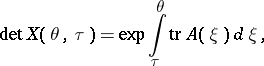where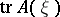is the trace of the operator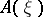.

The derivative of the Cauchy operatorof the system

at a point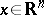is equal to the Cauchy operator of the system of equations in variations along the solutionof the system

the value of which atis(on the assumption that, for allin the interval with end pointsand, the graph oflies in a domain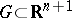such thatis a continuous mapping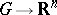with continuous derivative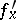in; this is one formulation of a theorem asserting the differentiability of the solution with respect to the initial value).

For a linear system (2) with constant coefficients (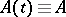), the Cauchy operator is defined by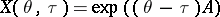(4)

(given a linear operator,is defined as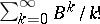; adopting a different approach, one can define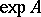via formula (4), putting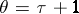). It is evident from (4) that the Cauchy operator depends only on the difference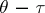of the parameters: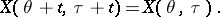This equation is a consequence of the autonomy of the system — a property valid for every autonomous system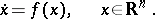(5)

Denoting the Cauchy operatorof the system (5) by, one obtains the following formulas from (3):For a linear system (2) with periodic coefficients, i.e.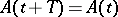for some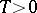and all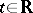, one has the identity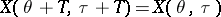for all; in this case the operator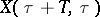, whereis arbitrary, is called the monodromy operator. The matrix defining the operator(or, say,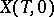) relative to some basis is called the monodromy matrix. All monodromy operators of a fixed linear system with periodic coefficients are similar to one another: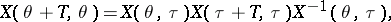therefore, the spectrum of the monodromy operatoris independent of. The eigen values of the monodromy operator are called the multipliers of the system; one can express conditions for stability and conditional stability of the system in terms of the multipliers (see Lyapunov characteristic exponent; Lyapunov stability; Stability theory). If the system (2) has periodic complex coefficients,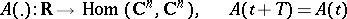for someand all, one has the theorem of Lyapunov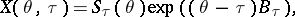where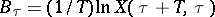, and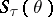is a non-singular linear operatorfor any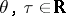, which is a periodic function of: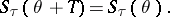Different names are sometimes used for the Cauchy operator (e.g. "matrizantmatrizant" for a linear system, or "operator of translation along trajectoriesoperator of translation along trajectories" ).

The operatordoes not usually have the name Cauchy attached to it in the Western literature, and in fact is usually not given any particular name at all. In Section 2.1 of [a2], Cauchy's role in the analysis of